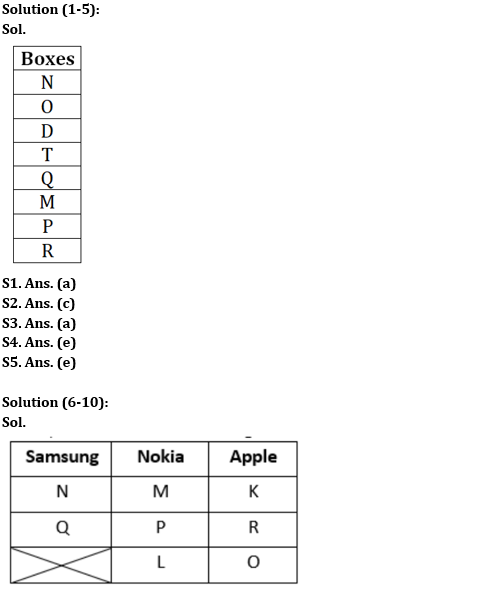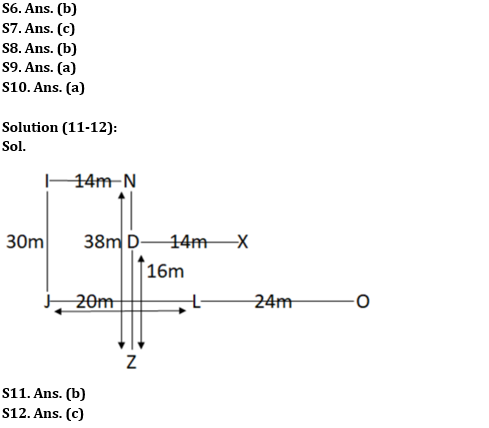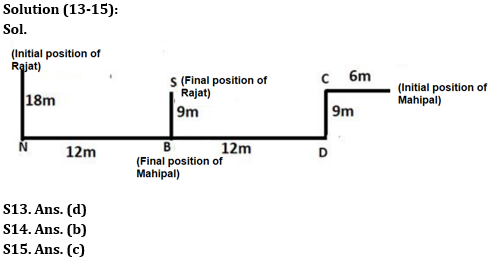Latest Banking jobs   »

# Reasoning Ability Quiz For IBPS RRB PO Prelims 2022- 16th June

Directions (1-5): Study the following information carefully and answer the questions accordingly.
There are at most 8 boxes that are kept one above another in a stack. Four boxes are kept between box M and box N which is kept either at the top or at the bottom position. Only one box is kept between box M and box R which is kept below box M. Three boxes are kept between box R and box T which is kept above box M. Box D is kept just below box O and not more than one box is kept above box O. Only one box is kept between box Q and box P which is kept just above box R.

Q1. How many boxes are there in the stack?
(a) Eight
(b) Seven
(c) Six
(d) Can’t be determined
(e) None of these

Q2. Which among the following box is kept at the bottom?
(a) N
(b) P
(c) R
(d) T
(e) M

Q3. Which among the following box is kept immediately above of box Q?
(a) T
(b) R
(c) N
(d) P
(e) None of the above

Q4. How many boxes are kept between box P and box N?
(a) One
(b) Four
(c) Three
(d) Two
(e) More than four

Q5. Four of the following five are alike in a certain way and hence form a group. Which is the one that doesn’t belong to that group?
(a) N and D
(b) Q and P
(c) T and M
(d) R and M
(e) P and R

Directions (6-10): Study the following information carefully and answer the questions accordingly.
Eight people K, L, M, N, O, P, Q, and R like either of the three phone brands i.e., Samsung, Nokia, or Apple. At least two and at most three of them like each brand. K does not like Nokia. M likes same brand as P. P neither likes Apple nor likes the same brand as K likes. Only two people like Samsung and one of them is N. Q does not like the brand which is liked by K and P. R neither likes the brand same as Q nor Nokia. L does not like the brand same as R.

Q6. Who amongst the following likes Nokia?
(a) Q
(b) L
(c) O
(d) R
(e) None of these

Q7. Who amongst the following likes the brand same as O?
(a) N
(b) Q
(c) K
(d) L
(e) None of these

Q8. Who amongst the following likes Samsung?
(a) N and O
(b) N and Q
(c) N and L
(d) N and R
(e) None of these

Q9. Which amongst the following statement is incorrect?
I. N and O like Samsung
II. L likes Nokia.
III. K, R and O like the same brand

(a) Only I
(b) Only II
(c) Only III
(d) Only I and II
(e) Only I and III

Q10. Four of the following five pairs are alike in a certain way and hence form a group. Which is the one that does not belong to that group?
(a) Q, O
(b) N, Q
(c) P, L
(d) R, O
(e) M, P

Directions (11-12): Study the following information carefully and answer the questions accordingly.
Point L is 24m west of point O. Point D is 14m west of Point X. Point L is 20m east of Point J. Point N is 38m north of Point Z. Point J is 30m south of Point I, which is 14m west of Point N. Point Z is 16m south of Point D.

Q11. Point D is in which direction with respect to point L?
(a) West
(b) North-West
(c) South
(d) North-East
(e) None of these

Q12. Four of the following five are alike in a certain way and hence form a group. Which is the one that does not belong to that group?
(a) I, D
(b) N, X
(c) O, N
(d) J, Z
(e) X, O

Directions (13-15): Study the following information carefully and answer the questions accordingly.
Two friends Rajat and Mahipal stand at different places on the ground. Rajat walked 18m towards the south direction and reached Point N, then turned towards his left and walked 12m to reach Point B, then turned towards his left and walked 9m to reach Point S. Mahipal walked 6m towards the west direction and reached Point C, then he turned towards his left and walked 9m to reach Point D, finally he turned towards his right and walked 12 m to point B and stopped.

Q13. What is the direction of initial position of Rajat with respect to initial position of Mahipal?
(a) South-west
(b) South-east
(c) East
(d) North-west
(e) None of these

Q14. What is the shortest distance between the final position of Mahipal and Point C?
(a) 12m
(b) 15m
(c) 20m
(d) 18m
(e) None of these

Q15. Which of the following points lie in the straight line?
(a) C and N
(b) C, D and S
(c) N, B and D
(d) S and N
(e) None of these

Solutions#### Congratulations!